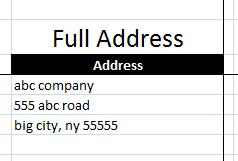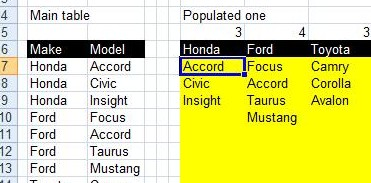## Walking Columns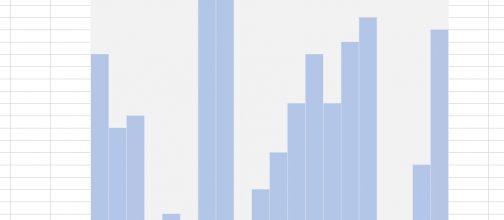An easy way to scroll through set of data in a graphic way without graphs. A-pure-formula xlsx workbook. These were done using formulas, conditional formatting, and ActiveX control.

## Sorting with functions only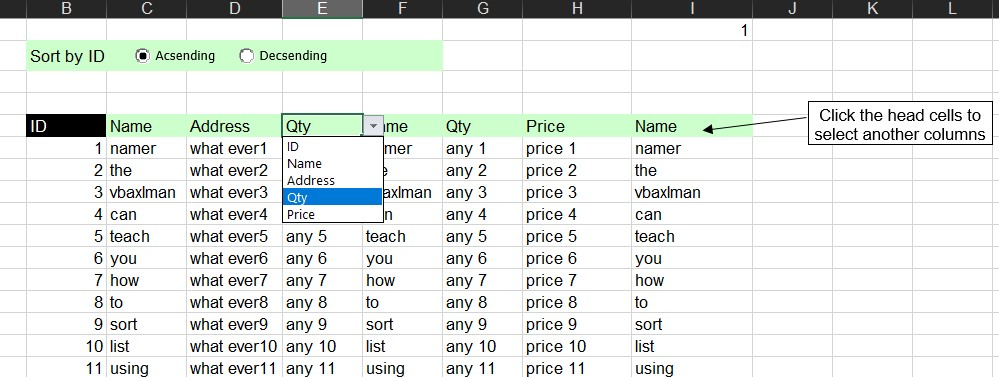This one is similar to http://xlfxs.com/sort-list-using-functions/ where we use some controls here that make it more user-friendly.

## Looplist – Repeating months using formulas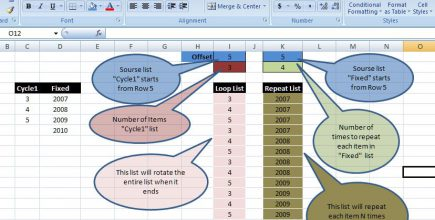Using formulas to repeat list, I call it LoopList Used to list Months for several years, in away that with 1 formula, it will loop through all months, then jumps …

## Get column name (columnname as A,B,C, etc) as input inside cell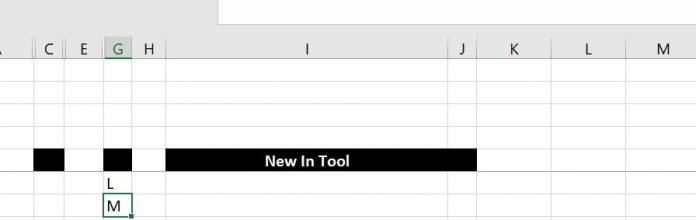Excel already has the formula ROW() =Row() Which as we may already know will give us the number of the row we are in, if you add no parameters However, …

## Fx to cut long column into two columns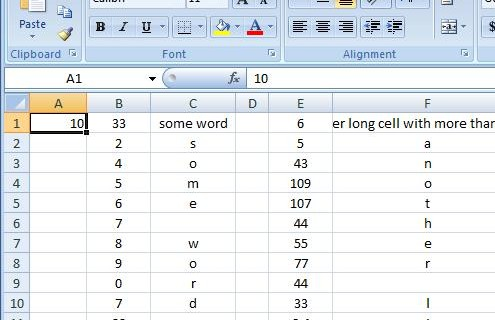Convert one long column into two This set of formulas will get the items after row 45 (for example) from column A into the column B starting from B1 to …